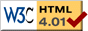# Billiards

previous next
• Sim
• Graph
• Time Graph
• Multi Graph

Physics-based simulation of 2D billiard balls, using the 2D Rigid Body Physics Engine. You can also regard this as air hockey pucks on a table.

Click near an object to exert a force with your mouse. Try changing gravity, elasticity (bounciness), and damping (friction).

Experiments to try:

• Check whether energy is conserved: turn on "show energy". You'll need to set damping=0 and elasticity=1.
• Try using different collision methods, such as "simultaneous". Is the simulation still realistic?

Note that this is a 2D simulation and doesn't treat important aspects of real world billiard balls such as friction and rotation or spin. The balls do not spin in this simulation because edge friction is not calculated, so collisions are essentially friction-less.

The method of collision handling for this simulation is important. Using the "simultaneous" method gives symmetric and non-realistic results. The linked article discusses why different methods of calculating the result of collisions are needed.

Also available are: open source code, documentation and a simple-compiled version which is more customizable.

previous next